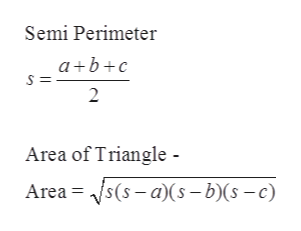# Find the area of a triangular garden if the sides are approximately 7 feet, 8 feet, and 14 feet (to the nearest square foot).

Question
8 views

Find the area of a triangular garden if the sides are approximately 7 feet, 8 feet, and 14 feet (to the nearest square foot).

check_circle

Step 1

Let the given sides of the triangles are a, b and c.

a = 7 feet

b = 8 feet

c = 14 feet

Step 2

Formula used:

Heron’s Formula:help_outlineImage TranscriptioncloseSemi Perimeter a bc 2 Area of Triangle - Area s(s-a)(5 - b)(s -c) fullscreen
Step 3

Semi-perimeter of the triang...

### Want to see the full answer?

See Solution

#### Want to see this answer and more?

Solutions are written by subject experts who are available 24/7. Questions are typically answered within 1 hour.*

See Solution
*Response times may vary by subject and question.
Tagged in

### Trigonometric Ratios